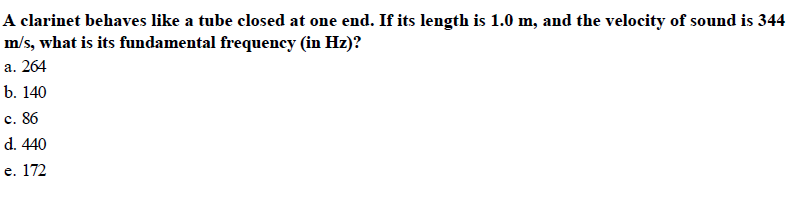A clarinet behaves like a tube closed at one end. If its length is 1.0 m, and the velocity of sound is 344m/s, what is its fundamental frequency (in Hz)?a. 264b. 140c. 86d. 440e. 172

Questionhelp_outlineImage TranscriptioncloseA clarinet behaves like a tube closed at one end. If its length is 1.0 m, and the velocity of sound is 344 m/s, what is its fundamental frequency (in Hz)? a. 264 b. 140 c. 86 d. 440 e. 172 fullscreen
Step 1

The fundamental frequency can be calculated as follows:

Given:

Step 2

Calculation:

The fundamental frequency of the ...

Want to see the full answer?

See Solution

Want to see this answer and more?

Our solutions are written by experts, many with advanced degrees, and available 24/7

See Solution
Tagged in

Wave Motion